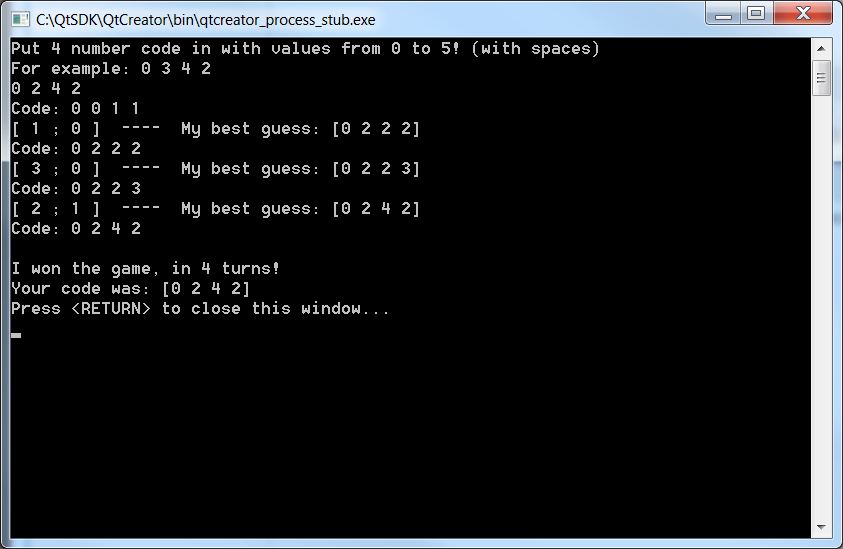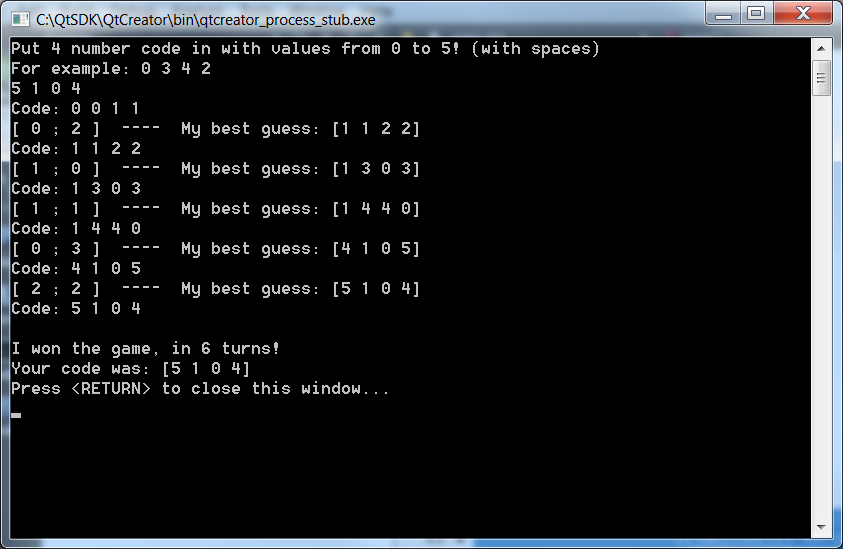## Automatic MasterMind Solver

For one of my university projects I had to find a way for automatically solving a mastermind game on a micro-controller in my case Arduino. There are several algorithms available on the internet, however most of them are very complicated or memory inefficient.

A very comon algorithm used to solve this problem is Knuth algorithm. Basically knuth algorithm stores all the possible cases in a array, and when the computer or the human makes the guess it eliminates all the cases which are not possible after the guess was made. It's a very good algorithm, however, it is very gready when it comes to memeory. It is physically impossible to put it on Arduino. So we had to find another solution.

After a lot of googling, I found Fran Van's gool algorithm, which was exactly what I was looking for. Simple, memory effiecient, fast in computation and reasonably low tries.

Bascially the algorithm just consists of a base 6 counter (depends on how many colors your version of game has). Initally you set the counter to something like 0000 or 0011 and make a guess. It will return you how many correct and misplaced pins you have. Save that result, and increment the counter. Afterwards check whether the new counter is consistent with all the previous guesses, if so save the result and increment the counter. You repeat this, until you find the code. If the counter overflows, goes back to 0000 or 0011, depends how you initialized, then there are no possible codes for the the correct and misplaced pins the user or someone else has given.

Anyway here is my implementation in C/C++:

#include
#include <time.h>
#include <stdio.h>
#include <stdlib.h>

using namespace std;
char input_code = {0}; //Code which needs to be cracked
char g_code = {0}; //code guessed by user or algorithm
char a_code = {0}; //code guessed by user or algorithm
char solution = {255}; //Holds the correct solutions
char checked = {0}; //With bits, holds information which color has been checked in corresponding slot
char incorrect = {255}; //Hold information which color was misplaced
char randNum; //just random number variable

//----------------------------------------------------------
// Function for returning the results of guess
//    0 - incorrect
//    1 - correct
//    2 - misplaced
//----------------------------------------------------------
void checkIfCorrect(char guess[], char result[], char code[])
{

result = 0;
result = 0;
char blacks = {0};
char whites = {0};

for(int i=0; i<4; i++)
{
if(guess[i] == code[i])
blacks[i]=1;
else
blacks[i]=0;
}

for(int i=0; i<4; i++)
{
if(blacks[i]!=1)
{
for(int f=0; f<4; f++)
{
if(f==i) continue;
if((guess[i] == code[f] && blacks[f] != 1))
if(whites[f] == 0)
{
whites[f]=1;
break;
}
}
}
}

for(int i=0; i<4; i++)
{
result += blacks[i];
result += whites[i];
}

}

int scoreCalculator(int black, int white)
{
if(black == 0 && white == 0) return 0;
if(black == 0 && white == 1) return 1;
if(black == 1 && white == 0) return 2;
if(black == 0 && white == 2) return 3;
if(black == 1 && white == 1) return 4;
if(black == 2 && white == 0) return 5;
if(black == 0 && white == 3) return 6;
if(black == 1 && white == 2) return 7;
if(black == 2 && white == 1) return 8;
if(black == 3 && white == 0) return 9;
if(black == 0 && white == 4) return 10;
if(black == 1 && white == 3) return 11;
if(black == 2 && white == 2) return 12;
if(black == 4 && white == 0) return 13;
}

void base6counter(char num)
{
num++;
if(num == 6)
{
num++;
if(num == 6)
{
num++;
if(num == 6)
{
num++;
if(num==6) num = 0;
num = 0;
}
num = 0;
}
num = 0;
}
}

int main()
{
srand(time(NULL));

//memset(g_code,0,4);
memset(solution,255,4);
memset(checked,0,4);
memset(incorrect, 255, 4);
cout << "Put 4 number code in with values from 0 to 5! (with spaces)" << endl << "For example: 0 3 4 2" << endl;
scanf("%d%d%d%d", &input_code, &input_code, &input_code, &input_code);

int guesses = 0;
char result[] = {0, 0};

g_code = 0; g_code = 0; g_code = 1; g_code = 1;
a_code = 0; a_code = 0; a_code = 1; a_code = 1;
char previous_scores;
while(guesses < 15)
{
guesses++;

cout << "Code: ";
//scanf("%d%d%d%d", &g_code, &g_code, &g_code, &g_code);
printf("%d %d %d %d",g_code,g_code,g_code,g_code);
//reset the misplaced variables

checkIfCorrect(g_code, result, input_code); //Check how if the guess was correct
int score = scoreCalculator(result,result);

previous_scores[guesses-1] = score;

if(result == 4)
break;

bool anySolutions = true;

while(anySolutions)
{
bool consistent = true;
for(int i=0; i<guesses; i++)
{
int test_score = scoreCalculator(result,result);
if(test_score != previous_scores[i])
{
consistent = false;
break;
}
}
if(consistent)
break;

base6counter(a_code);
if(a_code == 0 && a_code == 0 && a_code == 1 && a_code == 1)
{
anySolutions = false;
printf("ERROR: No solution possible!\n");
}
}

g_code = a_code;
g_code = a_code;
g_code = a_code;
g_code = a_code;

printf("\n[ %d ; %d ]  ----  My best guess: [%d %d %d %d]\n", result,result,a_code,a_code,a_code,a_code);

if(guesses == 8)
printf("\n-- %d %d %d %d -- \n",input_code,input_code,input_code,input_code);

}

if(result == 4)
{
cout << "\n\nI won the game, in " << guesses << " turns!" << endl << "Your code was: ";
printf("[%d %d %d %d]\n", g_code,g_code,g_code,g_code);
}
else
cout << "\n\nI\'m are crap in this game!" << endl;

return 0;
}

Some screen shots:• #### Implementing pulse oximeter using MAX30100

Mar 8, 2017 | by Raivis Strogonovs
• #### nRF51 Makefile with Qt Creator

Jun 4, 2016 | by Raivis Strogonovs
• #### USART, FreeRTOS and C++ on nRF51

Dec 14, 2015 | by Raivis Strogonovs
• #### Starting with nRF51 BLE and Qt Creator

Dec 12, 2015 | by Raivis Strogonovs
• #### Touch gesture recognition using body capacitance

Nov 29, 2014 | by Raivis Strogonovs
• #### Introduction to data encryption

Oct 4, 2014 | by Raivis Strogonovs
• #### MEMS (Part 2) – Guide to using gyroscope L3G4200D

Jun 17, 2014 | by Raivis Strogonovs